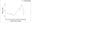# Refractive Index

SataSata

## Homework Statement

I have set up an experiment such that microwave is converged and beamed to a polymer slab and the reflected wave is measured. I have recorded angle of incidences from 15 to 90 degree and I found out that at 80 degrees, the output is the highest, while at 45 and 85 degrees, the output is the lowest. The thickness of the polymer slab, t is measured to be 5.2cm. Assuming the refractive index of air is 1.00, determine the order of interference m and the refractive index of the polymer slab, n.

## Homework Equations

Angle of Incidence = $\theta$
Optical Path Difference = OPD
Wavelength of Microwave = $\lambda$
Destructive Interference: $OPD = m\lambda =2t\sqrt(n^2 - (sin\theta)^2)$
Constructive Interference: $OPD = (m+1/2)\lambda =2t\sqrt(n^2 - (sin\theta)^2)$
EDIT: I come across another equation and is not sure if it's correct: $2t = \lambda/m$

## The Attempt at a Solution

I know that a 180 degrees phase shift happened here and assume that at angle of incidence = 80, the 2 beams which result from reflection and refraction must be exactly 180 degrees difference in phase hence OPD must be odd multiples of half of wavelength $\lambda$ to form a constructive interference.

While at either 45 or 85 degrees, the OPD must be integer multiples of wavelength to result in a destructive interference.

Hence I tried putting all the values in with 45 degrees in the destructive equation and 80 degrees in the constructive equation and form a pair of simultaneous equations.

However without the wavelength of the microwave, it can't be solved. I tried to assume the wavelength to be 0.01m but the resulting refractive index result to be lesser than air which is incorrect.

Am I missing something or is there something that I did not measure?

EDIT:
With the new equation $2t = \lambda/m$, I don't have to estimate the wavelength and after calculating 3 variables simultaneous equations:

I found n=2.008, m=1.371 and $\lambda$=14.26cm if I take $\theta=45$ for the destructive interference.

And n=1.0003, m=0.3004 and $\lambda$=3.124cm if i take $\theta=85$.

I'm not sure about m but I think both n and $\lambda$ are within acceptable values for $\theta=45$. Can anybody confirm? Which $\theta$ am I supposed to use anyway? Both 45 and 85 shows a drop in output. I have included a graph to show my measurements.

#### Attachments

•E2 Thin Film.jpg
5.6 KB · Views: 388
Last edited:

Homework Helper
•SataSata
SataSata
This is indeed like thin film interference. Just that the thin film is replaced with a polymer slab and the light waves are replaced with microwaves. I had revisited the lab and measured and calculated the wavelength of the microwave and solved the simultaneous equation with it.
However I was told to use the equations:
$I = I0 - I1cos(\theta - \theta min)$ to find the angle of incidence whereby the output is the lowest and excel derive it as 44.5 degrees as opposed to what I measured at 45 degrees.
$I = I2 + I3cos(\theta - \theta max)$ to find the angle where it is the highest which resulted in 78.95 degrees instead of what I measured as 80 degrees.
From what I understand, those 2 equations are from the double slit experiment whereby I'm not quite familiar with. Does anybody know how do I derive those 2 equations?
So I'm not supposed to use the values that I measured without using the 2 equations above due to the Taylor's theorem. Anybody know why?

Last edited:
Homework Helper
No, but it seems that these functions are generating symmetrical output values
around the minimum and maximum values - that is a cosine function is used to
give new output values. For some reason the microwave setup or interaction
with the material is distorting the output. I assume the new calculated output
values then displays a minimum and and maximum value at slightly different
angles. Apparently microwaves are absorbed at the rotational and torsional quantum
states of the molecules of the slab. It is difficult to hold quantum states (photons)
and interference (waves) together in one's thoughts!

•SataSata
SataSata
Another thing, I was told to measure the receiver output in voltage with a multi-meter instead of current. I was confused here as I thought that intensity should be proportional to current and the receiver clearly stated current as output. I have no idea how the receiver works but the transmitter look like a typical gunn diode transmitter. Voltage is supposed to show the potential difference so my guess is that the receiver measure the photoelectric effect. However shining light to the receiver shows no result. Can anybody confirm how voltage relate to the intensity and how the receiver works?

Homework Helper
Microwaves are part of the electromagnetic waves family.
That is they are transverse waves with varying electric and magnetic fields
propagating at the speed of light. They are transmitted, received and processed very
much like radio waves. Higher intensities registers as fields with larger amplitudes. Once
the microwave signal is converted to an electric signal the conversion between voltage
and current measurements can easily be achieved. If you give me larger current I can
easily convert it to a larger voltage and vice versa.
Very extensive site on all things microwaves related http://www.microwaves101.com/

Last edited:
•SataSata